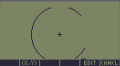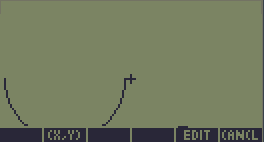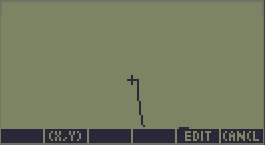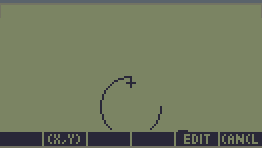Fast (& accurate) Arc circleFast (& accurate) Arc circle

Fast ArcDraws an arc circle into any Grob, or into Graphic Area if none provided.
Many Thanks to Cyrille de Brebisson for providing sample source code with many innovative ideas.

Fast Arc V1.0 : Binaries for HP48 S/SX/G/GX and HP49 G/G+, HP50G & HP48GII
Code Size : 895.5 Bytes; Checksum : HP48 : #933Eh; HP49 : #F119h

Input :
6 : Grob (<-- Optional, if none provided, will draw into Graphic Area)
5 : X0
4 : Y0
2 : Start Bearing (°)
1 : End Bearing (°)

Notes :
(0,0) is upper left.
Bearing convention is clockwise : 0° is North (or Front)
X0 & Y0 can be negative
All values are limited to 250.000 (Grob Width/Height, X0, Y0),
except Radius which is limited to 65.000.

Here follows some benchmark numbers :

 Name Input HP48SX HP48GX HP50G* 30pixels, Quarter circle X=65,Y=32,R=30,S=90,E=180 73ms 40ms 25ms 30pixels, Half circle X=65,Y=32,R=30,S=90,E=270 81ms 46ms 26ms 30pixels, Full circle X=65,Y=32,R=30,S=90,E=90 107ms 57ms 30ms 60pixels, Full circle X=30,Y=-10,R=60,S=90,E=90 125ms 75ms 33ms 150pixels, 3/4 circle X=65,Y=160,R=150,S=90,E=360 134ms 102ms 41ms
(*)Note : Thanks to Jacob Wall for providing HP50G results

PureCode version (without the header) show significant performance gains, as most of the time is in fact spent in the header (checking and reordering input for ASM code).
For 48GX, the 30pixels half-circle is in fact done in 14ms, while the quarter circle only needs 9ms. That means the header takes about 31ms for itself.
PureCode, however, is only usable for developers (too risky for end-user, see included doc).

This version is considered reasonably accurate.
For comparison with previous "approximative" versions, some screenshots follows :

30pixels half circle; starts and ends correctly
(X=32,Y=32,R=30,S=90,E=270)(X=68,Y=32,R=30,S=90,E=270)Large Half-circle : ends correctly
(X=168,Y=32,R=100,S=90,E=270)Three-Quarters small circle : ends correctly
(X=65,Y=44,R=15,S=90,E=0)Yann